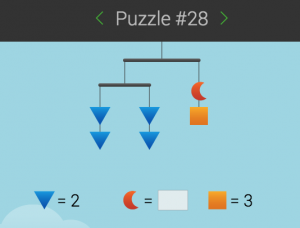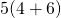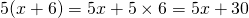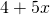# Getting Started: Solving Puzzles

SolveMeMobilesThe solve-me-mobiles puzzle page is a great place to start! Figure out an unknown number, and do it intuitively.

The job of algebra is to give us a way of writing or talking about unknowns while they are unknown.

In the image above, the unknown ‘weight’ is the moon shape. We could say, ‘how heavy is the moon shape when the mobile is balanced?’

You can figure it out with a little bit of multiply, dividing, adding, subtracting – the usual stuff.

That’s exactly what we do in algebra. Its just, we write the problem down in a lines of writing using numbers and letters which can be much more confusing than the nicely drawn diagram above. In fact, to transcribe the diagram above we might write,

Findwhengiven thatand.

Wa-at?

In early days in algebra, we tend to begin with only one letter to represent one unknown number. Our job is often to find out how much the unknown is worth – that’s called solving an equation. At other times we leave the letters in a formula because it represents a quantity that could change from day to day – such as the number of hours of daylight. Either way, the letters represent numbers, and can be treated as if they were numbers – we can multiply them, divide them, add to them, subtract from them and so on.

It may be that by the time we’re through with solving an equation we’ve applied many different arithmetic operations on the equation – sometimes we’re taught how to perform several steps at once (eg, ‘cross multiplication’, or ‘FOIL’). It’s quite easy to get lost in the world of algebra. Leave some breadcrumbs so that you can retrace your steps to begin thinking about algebra all over again. It takes time to make connections between ideas. The more you go over the ideas (especially if you are in the fortunate position of needing to explain an idea to someone else), the more the ideas fall into place with each other.

The word algebra comes from work written by Al-Khwarizmi.This page is taken from St Andrews University History of Math Website.

There have been lots of different ways to write about unknowns, however we’ve settled for an international math language that uses letters for unknown or variable quantities in equations, which behave just as if they were in fact visible to us as numbers.

For example:meansor, using the distributive law, it meansNow consider. We can’t evaluate it unless we find out what ‘someone somewhere’ wantsto be as a number. However, without that ‘someone somewhere’ we can still use the distributive law and say.

In other words, when we have unknowns we have some limitations, but we can keep going as if they were in fact known numbers by treating them with all the same rules.

Here are a couple of things that might not be obvious, and can cause confusion from day one:means four units plustimes whatever numberrepresents. When we put a number and a letter together, we mean that they are multiplied together.means four units plus. When we don’t put a number in front of a letter, it means that there is one of them!

The international language we use tries to achieve balance between clarity (having people understand it) and efficiency (writing as little as possible). That means that oftentimes, people can get left behind. Again, a little patience and retracing of steps, circling what doesn’t seem to make sense and having someone or a good resource explain can get a learner over these hurdles.